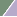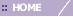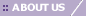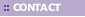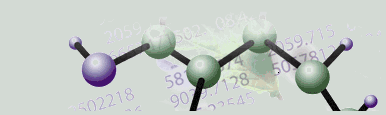# Overview of Principal Component Analysis (PCA) Functionality

Overview

Component Analysis is an unsupervised or class-free approach to finding the most informative or explanatory features in data. In particular, Principal Component Analysis (PCA) substantially reduces the complexity of data in which a large number of variables (e.g. thousands) are interrelated, such as in large-scale gene expression data obtained across a variety of different samples or conditions. PCA accomplishes this by computing a new, much smaller set of uncorrelated variables which best represent the original data. PCA is a powerful, well-established technique for data reduction and visualization. 2D and 3D PCA plots often place objects with similar patterns near each other.

GeneLinker™ provides one option for PCA analysis: Orientation by Genes or Orientation by Samples. In brief, PCA oriented by genes is useful for distinguishing sample classes or sample clusters, while PCA oriented by samples is useful for distinguishing gene classes or gene sets.

Mathematical Details and Examples of Orientation

To understand the difference and interpretive implications between the two different orientations - PCA by Genes or PCA by Samples - it is helpful to conceptualize the data analysis from the point of view of covariance matrices. A dataset can be thought of as comprising distinct mathematical or statistical variables (e.g. columns) for which there are statistical samples (e.g. rows).

a) Genes vs. Genes (Orientation by Genes)

• Typically, genes are considered the mathematical or statistical variables and samples are considered the statistical samples. The corresponding covariance matrix (if it were computed) would carry the covariance of one gene vs. another gene, assessed over the samples, and recorded for each pairwise combination of genes (i.e., pairwise combinations of the statistical variables). Thus, if there are n genes and m samples, the corresponding covariance matrix would comprise n by n entries, each entry being the covariance of the ith gene vs. the jth gene, i and j running from 1 through n. The ith element along the diagonal of this covariance matrix is simply the conventional variance of the ith variable, in this case the variance of the ith gene over all the m samples.

b) Samples vs. Samples (Orientation by Samples)

• However, if the samples are considered to be the mathematical or statistical variables, then the genes would play the role of the statistical samples. This case is less typical, but is still useful for biological interpretation in some situations (e.g., when the samples are different specific times of the cell cycle). In this case, the corresponding covariance matrix (if we were to compute it) would comprise m by m entries, each entry being the covariance of the ith sample vs. the jth sample from the data matrix. However, this time i and j run from 1 through m. Again, the ith element along the diagonal of this covariance matrix is simply the conventional variance of the ith variable. In this case, it is the variance of the ith sample (i.e., the ith mathematical or statistical variable) over all the n genes (the statistical samples).

In GeneLinker™, a Principal Component (PC) is defined as a mathematical entity (i.e., vector) computed from the data which is equivalent to a characteristic vector (i.e., eigenvector) of a covariance matrix derived from the data.

This is equivalent to finding the best lower dimensional linear basis set in which to represent the original data under the constraint of minimizing residual variance. The results obtained from the GeneLinker™ implementation are equivalent to a classical PCA of the data's covariance matrix; however, for computational speed and accuracy, covariance matrices are not explicitly computed by GeneLinker™ for PCA. From a covariance point of view, for example, a dataset typically comprises n genes by m samples. One can conceptualize two different kinds of covariance matrices for this data archetype:

a) Orientation by Genes: n by n covariance matrix (genes in the role of the math/statistics variables; hence, n genes vs. n genes, aggregated over all samples) OR

b) Orientation by Samples: m by m covariance matrix (samples in the role of the math/statistics variables; hence, m samples vs. m samples, aggregated over all genes).

For example, if there are n=1000 genes and m=12 samples (12 different human subjects, for example), the covariance matrix for case (a) would have 1000000 elements (1000 x 1000), but the covariance matrix for case (b) would have only 144 elements (12 x 12).

Technical Notes

Whether PCA orientation by genes or by samples, the maximum number of bona fide Principal Components that can be returned is the smaller of the number of genes or the number of samples. This is an inherent mathematical constraint.

PC calculation does not require parameters, and none are set by you beyond selecting the orientation of the calculation. The PCA Components to Display setting in the Preferences (accessed from the Edit menu) only affects display and reporting. The default limit on the number of PCs displayed in the Scree and Loadings plots is 15. This setting does not affect the actual calculation of the PCs. It sets an upper limit only on the number of PC's to display in these plots; therefore it does not have to be set before the PCs are calculated.

Whether the user requests PCA of count data, log data, max-min normalized data, missing value-replaced data, etc., GeneLinker™ automatically zero-means the data 'variables' before the PCA calculation, as is required for the results to be mathematically equivalent to the PCA of the covariance matrix.

GeneLinker™ limits the number of PCs by their contribution towards representing fractions of the total variance of the date (i.e., their numerical relevance). Only PCs associated with respective eigenvalues greater than or equal to 10-8 are included in the calculation result set. But in practice PCs with respective eigenvalues (i.e., fractions of data total variance) less than about 0.1 are rarely of much interpretive use or value.

Note also that a PC's pointing direction (e.g., southeast rather than northwest) along the line co-linear with the PC is irrelevant. Therefore, reversing the algebraic signs of all the constituent values of a PC in, for example, a Loadings Line Plot, is irrelevant.

Related Topics:

Performing PCA for a Dataset

Creating a 3D Score Plot

Tutorial 5: Principal Component Analysis (PCA)